Structural devices

This free course is available to start right now. Review the full course description and key learning outcomes and create an account and enrol if you want a free statement of participation.

Free course

# 6.3.1 Damped harmonic oscillator

Starting once again with Newton's second law but including the additional damping force in the equation gives: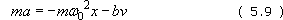## SAQ 10

Where did the term ω02 in Equation (5.9) come from?

### Answer

The term ω02 comes from rearranging Equation (5.5). This is due to the solution that I identified for the simple (undamped) harmonic oscillator, x = A cosω0t. Remember that ω0 is the natural angular frequency of the system.

Transposing and writing this expression in differential form I have: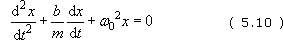Remember that dx/dt = v, the velocity.

As with the simple harmonic oscillator, we can solve this equation for x as a function of t. This time there are three solutions and they depend on the values of the constants.

Before you look at these solutions answer the following question.

## SAQ 11

The effect of damping is bound to make the oscillations die away. Imagine holding one end of the spring of a mass-spring system and setting it into oscillation:

• (a) What would you expect the influence of a large damping coefficient to be on the rate of decay?

• (b) What would you expect the influence of a large mass to be on the rate of decay?

### Answer

• (a) A large damping coefficient would imply that the losses are high, so I would expect the oscillations to decay quickly.

• (b) A large mass will resist the damping more readily (think of a heavy mass on a spring; it will be less affected by the surrounding air) and so it will tend to reduce the rate of decay.

It is the value of b/m that is significant in identifying how the system will behave. In the extreme, where this value is very small (large mass or small damping coefficient) the system will behave like the undamped system described earlier and oscillate indefinitely.

You can see that, in this case, the velocity-dependent term in Equation (5.10) will disappear as b/m tends to zero and the equation will be identical to Equation (5.7). On the other hand, if b/m is very large then this term will dominate and, as you will see, the system will not oscillate at all.

And now to the three solutions.

1. The underdamped case

The underdamped case occurs when ω0 > b/2m. As in the previous case, the system oscillates but now, as expected, the amplitude decays. The solution takes the form: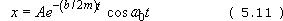Again, I have ignored the phase term. The more general solution is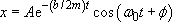but an appropriate choice of start time enables me to set φ to zero.

The amplitude term Ae−(b/2m)t will decay with time; the minus sign in the exponent combined with large values for t (as time goes on) ensures this. The rate of decay will depend on the value of b/m. If this value is large, then the decay will be rapid. This corresponds to a large damping force or a small mass. See Figure 25(a).

2. The overdamped case

The overdamped case occurs when ω0 < b/2m. Now the system doesn't oscillate at all; the motion simply dies away. This is characterised by a solution which decays exponentially: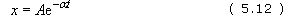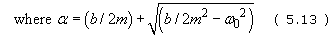The important thing to notice here is that there are no oscillations; you can see this because there is no cosine term. See Figure 25(b).

3. The critically damped case

The critically damped case occurs when ω0 = b/2m. The solution has the form: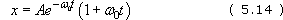This is a special case where the displacement goes to zero at the fastest possible rate. Clearly, again, there are no oscillations. You can think of this as the smallest value of b/m before the onset of oscillatory behaviour. See Figure 26(c).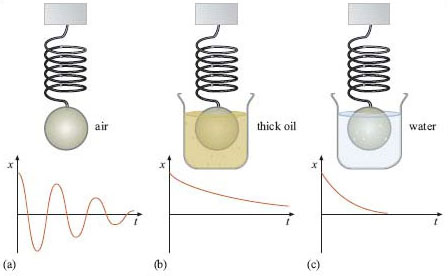Figure 26 Plots of displacement vs time for the mass-spring system: (a) underdamped – mass in air; (b) overdamped – mass in thick oil; (c) critically damped – mass in water

As with the solution for the simple harmonic oscillator (SHO), you can check these results by differentiating the solutions above and inserting them into the appropriate places in Equation (5.10).

## SAQ 12

If I want to engineer a damped mass-spring system to have a high natural frequency and a rapid decay rate, what physical properties should I prescribe?

### Answer

There are three physical parameters for which I can choose the properties: the spring constant, the mass and the damping coefficient. The natural period is proportional to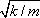so I should choose either a large value for the spring constant or a small mass. On the other hand, the decay rate is inversely proportional to b/m. So I need either a small damping coefficient or a large mass. In practice, choosing which of the above to alter will depend on the options that I have available.

T356_1

### Take your learning further

Making the decision to study can be a big step, which is why you'll want a trusted University. The Open University has 50 years’ experience delivering flexible learning and 170,000 students are studying with us right now. Take a look at all Open University courses.

If you are new to university level study, find out more about the types of qualifications we offer, including our entry level Access courses and Certificates.

Not ready for University study then browse over 900 free courses on OpenLearn and sign up to our newsletter to hear about new free courses as they are released.

Every year, thousands of students decide to study with The Open University. With over 120 qualifications, we’ve got the right course for you.

Request an Open University prospectus